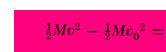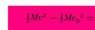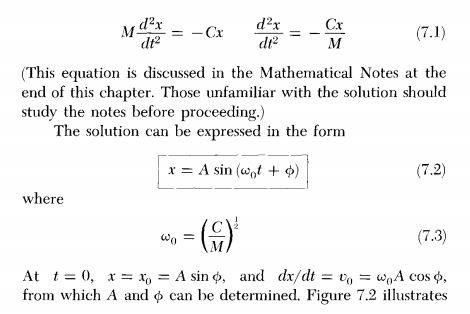# Oscillators and conservation of energy

velvetmist
In the equation 7.4, the author is taking v0=√(C/M)*x, and I don't get where does that come from. I would really appreciatte your help, thanks.

#### Attachments

Mentor
Could you please define the terms in the equation you posted? This is for a spring oscillator, right? Thanks.

Can you say what you think is being expressed with this equation? What is the total energy of a simple spring oscillator made up of?

Mentor
In the equation 7.4, the author is taking v0=√(C/M)*x
And you posted equation 7.5.

velvetmist
And you posted equation 7.5.
Sorry, I made a typo, I posted the correct one.

What is the total energy of a simple spring oscillator made up of?
Yes.

This is for a spring oscillator, right?
Yes.

Could you please define the terms in the equation you posted?
It doesn't say much, cause it's trying to explain spring oscillators in a general way.

#### Attachments

Staff Emeritus
Gold Member
v0=√(C/M)*x

Maybe I missed it, but I don't see this in any of the stuff you posted.

velvetmist
Maybe I missed it, but I don't see this in any of the stuff you posted.
If you do the integral you got:. Then -M*v02=C*x2. So v0=√(-C/M)*x.

Edit: i just realized i forgot to put the minus.

#### Attachments

Last edited:
Homework Helper
If you do the integral you got: View attachment 231123. Then -M*v02=C*x2. So v0=√(-C/M)*x.

Edit: i just realized i forgot to put the minus.
If you are going to post typeset equations, it would be worthwhile to learn a little LaTeX. Info => HowTo => https://www.physicsforums.com/help/latexhelp/

For example, $$\frac 1 2 Mv^2 - \frac 1 2 Mv_0^2$$

velvetmist
If you are going to post typeset equations, it would be worthwhile to learn a little LaTeX. Info => HowTo => https://www.physicsforums.com/help/latexhelp/

For example, $$\frac 1 2 Mv^2 - \frac 1 2 Mv_0^2$$

If you do the integral you got:. Then -M*v02=C*x2. So v0=√(-C/M)*x.

$$-M v^2_0 = C x^2 .$$ Therefore $$v_0 = \sqrt {\frac {-C} {M}} x .$$

Btw, the text defines ##w_0 = \sqrt {\frac {C} {M}}, v_0 = w_0 A \cos(\phi). ## Then if ##v_0 = \sqrt {\frac {-C} {M}} x, x = A \cos(\phi). ## But we got that ## x = A \sin(\phi),## so ##\cos(\phi)## would be equal to ##\sin(\phi)## and that's not true. And even if it were true, i'm not taking into account that ##w_0 = \sqrt {\frac {C} {M}},## and not equal to ##\sqrt {\frac {-C} {M}}.##

#### Attachments

Staff Emeritus
Gold Member
Okay, now that I have figured out that I have a copy of the text (used in the first mechanics course I took all those decades ago), maybe we can have a discussion.

I don't understand what you mean when you write

If you do the integral you got ##\frac{1}{2} Mv^2 - \frac{1}{2} Mv_0^2 =##.

Do what integral? Also, this only half of an equation!

Then ##-M v^2_0 = C x^2##

I don't see how you got this.

Equation (7.5) in the text is
$$\frac{1}{2} Mv^2 + \frac{1}{2} Cx^2 = \frac{1}{2} M \left( \frac{dx}{dt} \right)^2 + \frac{1}{2} Cx^2 = E,$$
where ##E## is the constant ##\frac{1}{2} CA^2##. Here, ##C## is the spring constant and ##A## is the amplitude of oscillation..

This means that
$$\frac{1}{2} Mv^2 + \frac{1}{2} Cx^2 = \frac{1}{2} CA^2$$
at all times ##t##. Setting ##t=0## gives
$$\frac{1}{2} Mv_0^2 + \frac{1}{2} Cx_0^2 = \frac{1}{2} CA^2,$$
so that
$$v_0^2 = \frac{C}{M} \left( A^2 - x_0^2 \right) = \omega_0^2 \left( A^2 - x_0^2 \right).$$

Also, I do not understand

But we got that ## x = A \sin(\phi)##

Actually, ##x = A \sin \left( \omega_0 t + \phi \right)##.

velvetmist
Do what integral? Also, this only half of an equation!
(5.12) integral. And yes, i just realized that I cropted it in a wrong way, but basically: $$\Delta T = \frac 1 2 Mv^2-\frac 1 2 Mv_0^2.$$

I don't see how you got this.
Cause we have that
$$\Delta T = \frac 1 2 Mv^2-\frac 1 2 Mv_0^2 = \frac 1 2 Mv^2-\frac 1 2 Cx^2,$$
and that's only true if ##−Mv_0^2=Cx^2.##

Actually, ##x = A \sin \left( \omega_0 t + \phi \right)##.
I'm just taking that from the text book. I already posted that part but i will do it again if that helps u:#### Attachments

Staff Emeritus
Gold Member
Cause we have that
$$\Delta T = \frac 1 2 Mv^2-\frac 1 2 Mv_0^2 = \frac 1 2 Mv^2-\frac 1 2 Cx^2,$$

I agree with
$$\Delta T = \frac 1 2 Mv^2-\frac 1 2 Mv_0^2 ,$$
but I do not understand the second equals sign, I do not understand why
$$\Delta T = \frac 1 2 Mv^2-\frac 1 2 Mv_0^2$$
is also equal to
$$\frac 1 2 Mv^2-\frac 1 2 Cx^2 .$$

I'm just taking that from the text book.

No, you have not taken it from the textbook. You wrote (and used)

But we got that ## x = A \sin(\phi)##

which is incorrect, and which is not in the textbook.

velvetmist
I agree with
$$\Delta T = \frac 1 2 Mv^2-\frac 1 2 Mv_0^2 ,$$
but I do not understand the second equals sign, I do not understand why
$$\Delta T = \frac 1 2 Mv^2-\frac 1 2 Mv_0^2$$
is also equal to
$$\frac 1 2 Mv^2-\frac 1 2 Cx^2 .$$
Sorry, sorry, I'm really sorry, i just made a lot of typos, I meant
$$\frac 1 2 Mv^2+\frac 1 2 Cx^2 .$$
That's because
$$\Delta (T+V) =\Delta E = \frac 1 2 Mv^2-\frac 1 2 Mv_0^2,$$
cause here ##\Delta V=0.## And we also know that
$$\frac{1}{2} Mv^2 + \frac{1}{2} Cx^2 = \frac{1}{2} M \left( \frac{dx}{dt} \right)^2 + \frac{1}{2} Cx^2 = E,$$
then
$$\frac 1 2 Mv^2-\frac 1 2 Mv_0^2 = \frac{1}{2} M \left( \frac{dx}{dt} \right)^2 + \frac{1}{2} Cx^2.$$

No, you have not taken it from the textbook. You wrote (and used)
Sorry, i just didn't read "at t=0", which is a huge mistake, but even like that i think is kind of weird cause he have that
$$v_0 = \sqrt {\frac {C} M} A \cos(\phi) = \sqrt {\frac {-C} M}x .$$
And we know that
$$x = A \sin \left( \omega_0 t + \phi \right).$$
Then
$$v_0 = \sqrt {\frac {C} M} A \cos(\phi) = \sqrt {\frac {-C} M} A \sin \left( \omega_0 t + \phi \right).$$
$$\sqrt {-1}\cos(\phi)=\sin \left( \omega_0 t + \phi \right).$$
I probably made a mistake, or something like that cause i find this equation really weird.

Staff Emeritus
Gold Member
That's because
$$\Delta (T+V) =\Delta E = \frac 1 2 Mv^2-\frac 1 2 Mv_0^2,$$
cause here ##\Delta V=0.##

No,
$$\Delta V = \frac{1}{2} C x^2 - \frac{1}{2} C x_0^2 .$$

See equation (5.20).

•velvetmist
velvetmist
No,
$$\Delta V = \frac{1}{2} C x^2 - \frac{1}{2} C x_0^2 .$$

See equation (5.20).
Thank you so much! I finally get it, all of my questions were between pages 149 and 150. We will see conservation of energy in three classes, that's why I was so confused about that.